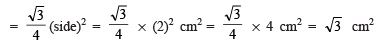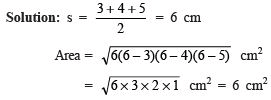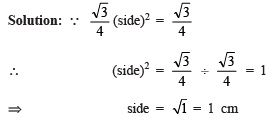Very Short Answers Type Questions- Heron’s Formula

# Very Short Answers Type Questions- Heron’s Formula - Notes | Study Mathematics (Maths) Class 9 - Class 9

 1 Crore+ students have signed up on EduRev. Have you?

Question 1. What is the area of an equilateral triangle whose side is 2 cm?
Solution:
Area of an equilateral triangleQuestion 2. Find the area of a triangle whose sides are 3 cm, 4 cm and 5 cm.Question 3. If the area of an equilateral is √3/4 cm2 then find the side of the triangle.The document Very Short Answers Type Questions- Heron’s Formula - Notes | Study Mathematics (Maths) Class 9 - Class 9 is a part of the Class 9 Course Mathematics (Maths) Class 9.
All you need of Class 9 at this link: Class 9

## Mathematics (Maths) Class 9

88 videos|397 docs|109 tests
 Use Code STAYHOME200 and get INR 200 additional OFF

## Mathematics (Maths) Class 9

88 videos|397 docs|109 tests

Track your progress, build streaks, highlight & save important lessons and more!

,

,

,

,

,

,

,

,

,

,

,

,

,

,

,

,

,

,

,

,

,

;